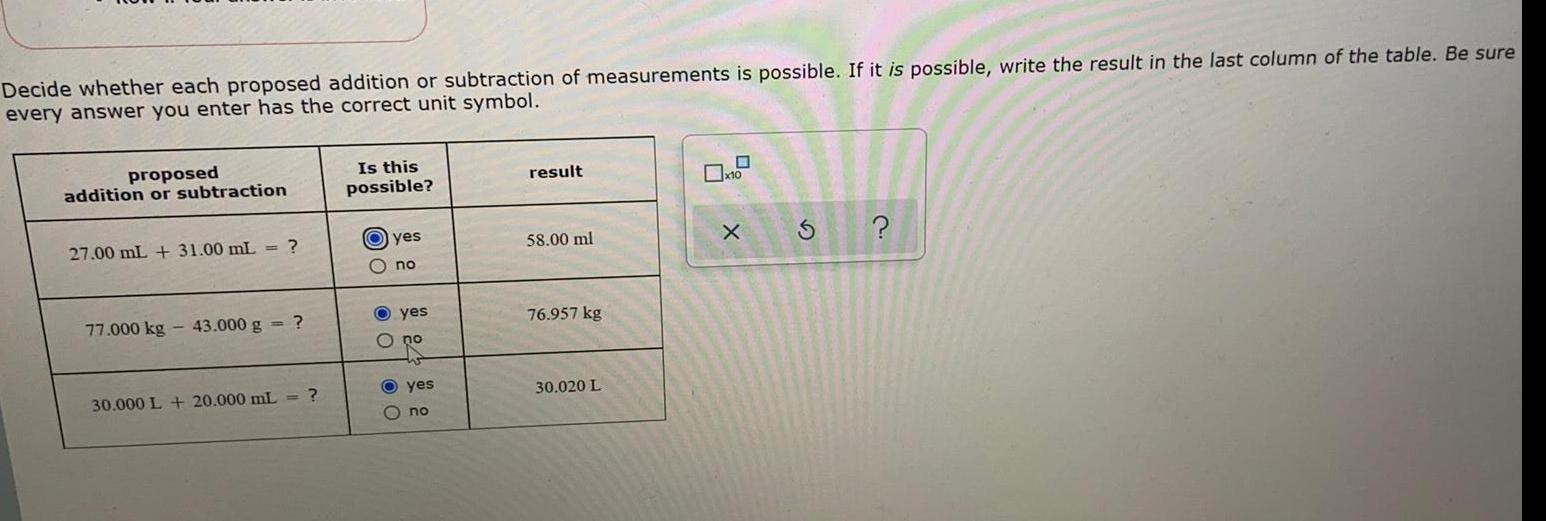Question:

# Decide whether each proposed addition or subtraction ofDecide whether each proposed addition or subtraction of measurements is possible. If it is possible, write the result in the last column of the table. Be sure every answer you enter has the correct unit symbol. proposed addition or subtraction 27.00 mL + 31.00 mL = ? 77.000 kg - 43.000 g = ? 30.000 L + 20.000 mL = ?